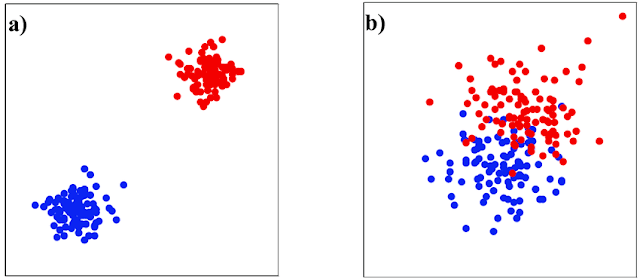# Intra class vs Inter class correlation in statistics

Interclass correlation and intra-class correlation are two special cases of correlation analysis. Interclass correlation shows the association among the groups and intra-class correlation shows the association within a group.Intra class(a) vs Inter class(b)

“Interclass” means among the groups.
“Intra class” means within group.

### Interclass correlation

The interclass correlation means the relationship among the groups of data or between the groups of data. For example-
suppose we have two groups of data named height and weight. when we build up a relationship between that two groups then this relation is called inter class correlation. And the coefficient which define their relationship is called the coefficient of inter class correlation.
The pearson correlation coefficient is used to measure inter class correlation.

### Intra class correlation

On the other hand intra class correlation means the relationship within data group. For example-
Suppose we only one group of data named height. When we build a relationship among or between the data of height group then this relation is called intra class correlation. And the coefficient which define the relation is called the coefficient of intra class correlation.

### Application of Intra class correlation

Intraclass correlation used to measure a wide variety of numerical data from clusters or groups, including:
• How closely relatives resemble each other with regard to a certain characteristic or traits.
• Reproducibility of numerical measurements made by different people measuring the same thing.

From the above discussion now it is very clear about intra class correlation and interclass correlation. I think there are no confusion about inter class and intra class correlation.

Try some fresh contents: